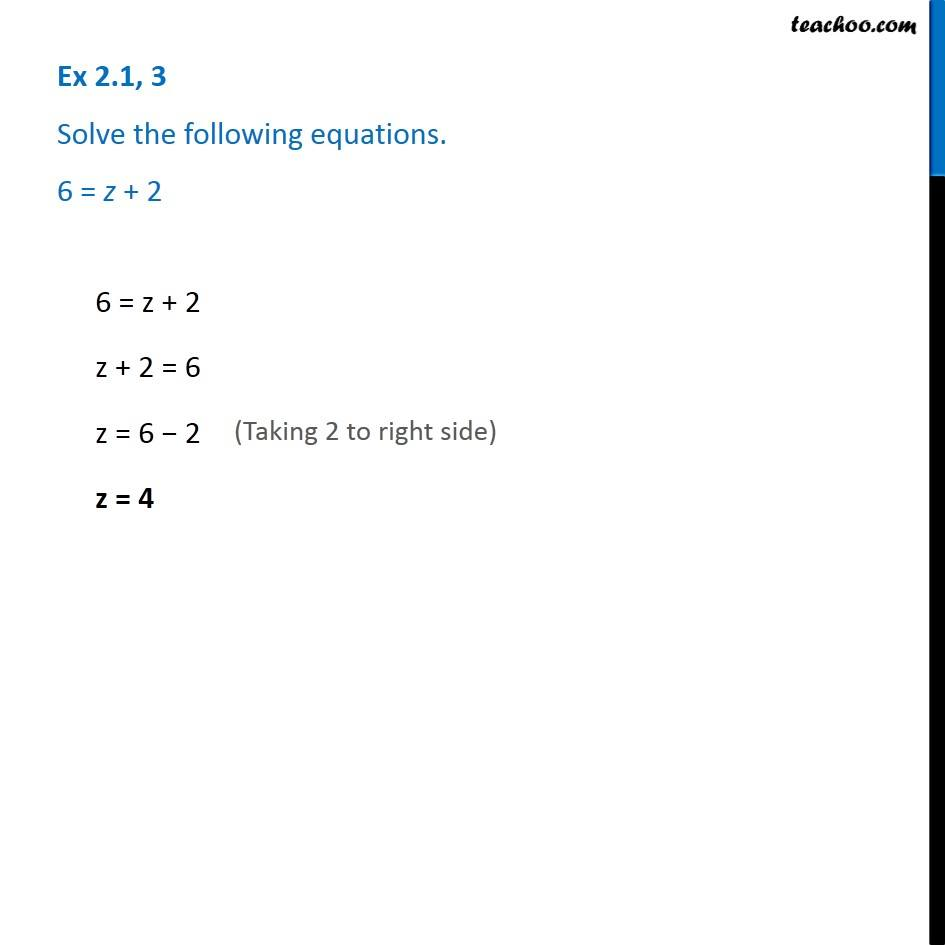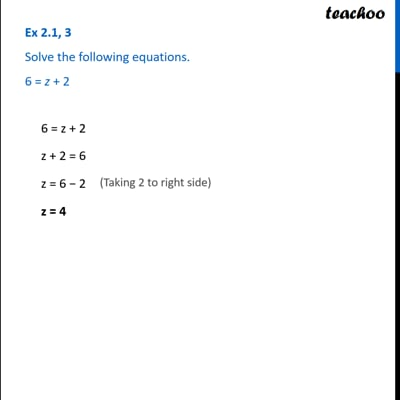Solving easy equations

Chapter 2 Class 8 Linear Equations in One Variable
Serial order wiseThis video is only available for Teachoo black users

Learn in your speed, with individual attention - Teachoo Maths 1-on-1 Class

### Transcript

Question 3 - Chapter 2 Class 8 - Linear Equations in one variable - NCERT Book Solve the following equations. 6 = z + 2 6 = z + 2 z + 2 = 6 (Taking 2 to right side) z = 6 − 2 z = 4